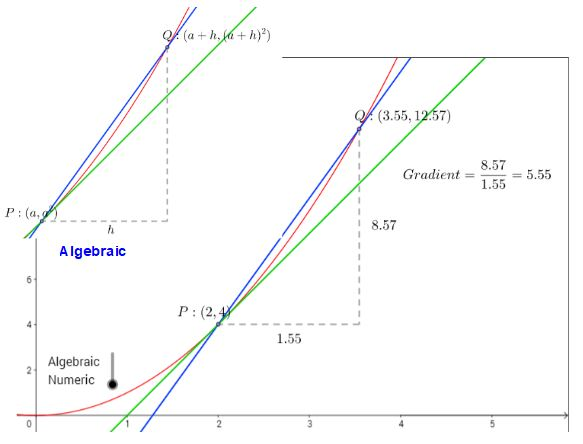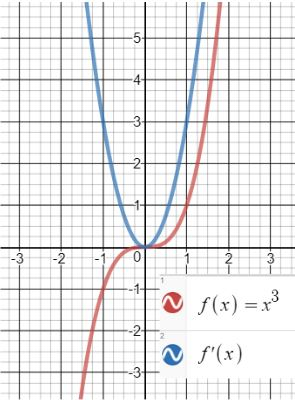# Formulae: A Level Mathematics

Looking at the Subject content for A Level Mathematics we see that Appendix A, pages 16-22 describes the mathematical notation for AS and A level qualifications in mathematics and further mathematics. Appendix B, pages 23-26 is on mathematical formulae and identities.Checking individual examination board specifications shows us the formulae which will be provided in the examination; each looks very similar.OCR (MEI) Formulae

I think it is useful for students to be aware as they study the course which formulae they must know and which will be provided; though they should be very familiar with any provided formulae.

Teaching Calculus from the new specifications I see that the formula for Differentiation from first principles is provided which seems fair. Looking at MEI’s very helpful advice on Integrating Technology into your scheme of work we see some suggested resources for teaching differentiation, including this GeoGebra resource on First Principes. I like the way one can choose between numeric and algebraic.MEI – Use of Technology, Differentiation

Staying with Calculus and technology, note that Desmos allows you to very easily see a function and its gradient function; note the requirement of the subject content that students should be able to sketch the gradient function for a given curve.A resource I found very useful for the matching a functions with their gradient functions comes from Underground Maths. I included Gradient Match which can be used interactively online in this post on introducing gradients at GCSE. Note that you can simplify the task by giving students the set of six functions and the six gradient functions separately.